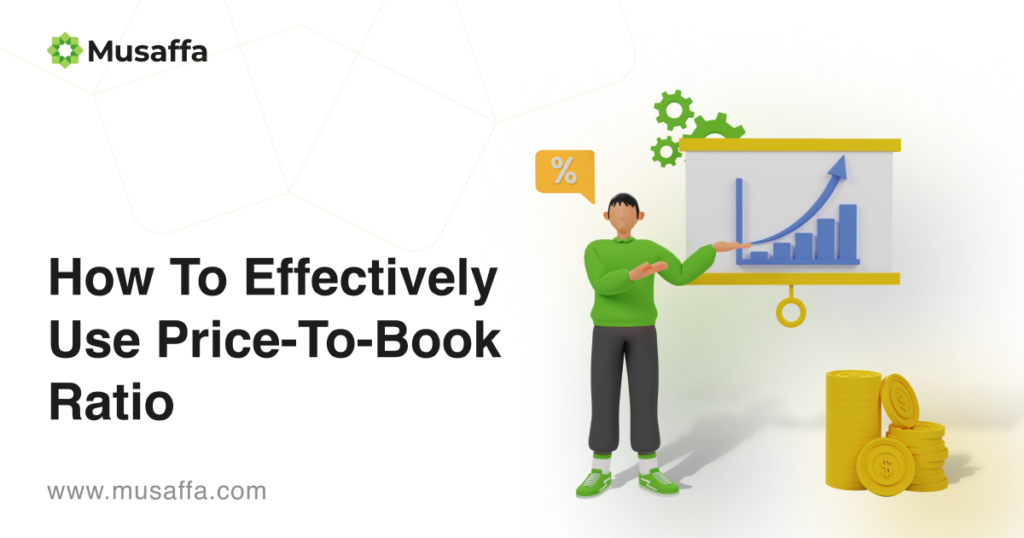# How To Effectively Use Price-To-Book Ratio#### What Is the price-to-book ratio?

The price-to-book (P/B)ratio is vital to value investors because it signals that the stock is selling less than its intrinsic value. It shows investors whether the company is overvalued or undervalued. Thus, the P/B ratio that is lower than one may signal that the current stock is undervalued. On the other hand, a P/B ratio greater than one may signal that a stock is overvalued. The price-to-book ratio (P/B) metric can help investors avoid overvalued companies and find undervalued companies.

#### What is a good price-to-book ratio?

Generally, when the P/B value is close to 1, it means that the stock is trading at near-book value. P/B value of 0.5 means that the stock is trading at half the book value. This can be a good opportunity for investors to buy and wait until the market adjusts to a higher price. On the other hand, a P/B ratio of 3 or higher might signal that a market value is already high and may expect a fall. However, it is difficult to identify a single standard for “good” P/B ratios because every industry has a different range; thus, what’s high in one industry may be low in another sector. Of course, this ratio has its limitations, and it may not be the most influential metric for valuation.

#### Example of how to calculate price-to-book ratio

Let’s calculate the P/B ratio for Company A. And let’s say that Company A has the following data:

Total assets = \$5 billion

Total liabilities = \$4 billion

Outstanding shares = 100 million

Stock price = \$20

First, we calculate Company A’s book value by subtracting \$4 billion (liabilities) from \$5 billion (assets). So, the book value becomes \$1 billion.

• Book value= \$1 billion (\$5billion – \$4billion).

In the second step, to find the per-share book value, we divide \$1 billion by the 100 million outstanding shares.

• Per-share book value= \$10 (\$1billion/100million shares).

Finally, to find out the price- to book value, we divide the current share price of \$20 by a per-share book value of \$10.

• Price to Book ratio = 2 (\$20 / \$10).

In simple terms, we can say that the stock is trading at two times higher than its book value.

As an investor, it would be wise if you track company A’s price-to-book ratio over some time and watch if it’s holding steady, increasing, or falling.

You can also compare company A to another company in the same industry. If company B has a higher P/B ratio (e.g., 4), it could signal that the market expects strong earnings growth and is bidding up the shares in anticipation. You can conclude that B company was already overvalued. On the contrary, if company B had a P/B of 0.5, you might have supposed that the stock price is trading at a discount because investors are paying below book value for it.